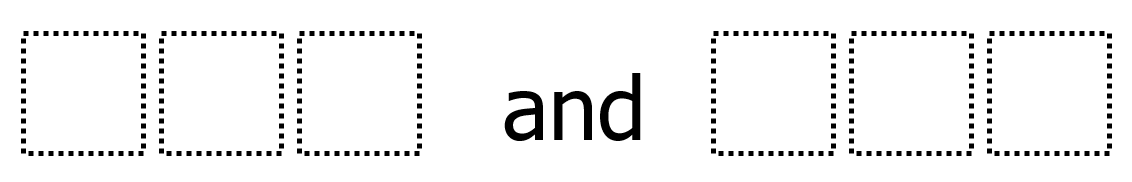# Rounding 1

Directions: Using the digits 0 to 9 at most one time each, place a digit in each box to make two different three-digit numbers that round (to the nearest hundred) to 500.### Hint

What’s a number that definitely will NOT round (to the nearest hundred) to 500? How can you have a number that is less than 500 round to 500? How can you have a number that is greater than 500 round to 500?

There are many possible answers, but one will be in the range of 460 to 498 and the other will be in the range of 501 to 539.

Source: Robert Kaplinsky

## Multiply and Divide Within A Hundred 1

Directions: Using the digits 2 to 9 at most one time each, place a digit …

### One comment

1.455 and 523